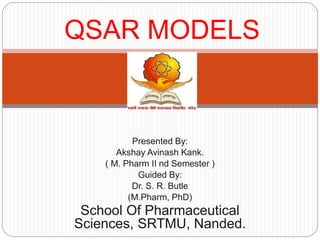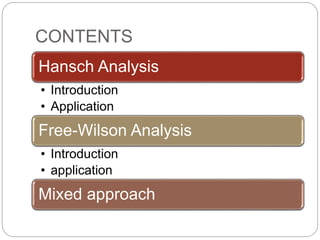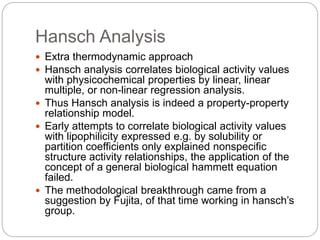Successfully reported this slideshow.

# Hansch and Free-Wilson QSAR Models×

1 of 23 Ad
1 of 23 Ad

# Hansch and Free-Wilson QSAR Models

In this slide I covered the detailed about hansch analysis, Free-Wilson analysis, and Mixed approach. I also gave a detailed application for each points.

In this slide I covered the detailed about hansch analysis, Free-Wilson analysis, and Mixed approach. I also gave a detailed application for each points.

## More Related Content

### Hansch and Free-Wilson QSAR Models

1. 1. Presented By: Akshay Avinash Kank. ( M. Pharm II nd Semester ) Guided By: Dr. S. R. Butle (M.Pharm, PhD) School Of Pharmaceutical Sciences, SRTMU, Nanded. QSAR MODELS
2. 2. CONTENTS Hansch Analysis • Introduction • Application Free-Wilson Analysis • Introduction • application Mixed approach
3. 3. Hansch Analysis  Extra thermodynamic approach  Hansch analysis correlates biological activity values with physicochemical properties by linear, linear multiple, or non-linear regression analysis.  Thus Hansch analysis is indeed a property-property relationship model.  Early attempts to correlate biological activity values with lipophilicity expressed e.g. by solubility or partition coefficients only explained nonspecific structure activity relationships, the application of the concept of a general biological hammett equation failed.  The methodological breakthrough came from a suggestion by Fujita, of that time working in hansch’s group.
4. 4. Hansch Analysis  Hansch and Fujita combined different physico- chemical parameters in one equation.  Where, C – Molar concentration k1, k2, k3, – Coefficients determined by least squares procedure  For in vivo parabolic lipophilicity values in included in above equation, hence it becomes
5. 5. Hansch Analysis  Later to this, steric parameters were added to this general model and even latter molar refractivity values.  With such multiparameter equation it was possible to describe much more complex dependence of biological activities on physico- chemical properties.  In the last three decades nearly all conceivable combinations of lipophilic, polarizability, electronic and steric parameters with or without additional indicator variables have been used and correlated with biological activity values in linear, parabolic and bilinear equation.
6. 6. Application  Hansch analysis can be used to describe complex biological data, where several different transport processes and equilibria contribute to the overall structural activity relationship.  However, in industrial practice such relationships and their quantitative description are of almost importance.  Instead of wasting thousands of animals nowadays enzyme inhibition, receptor binding and cell culture data are used to derive the activity profiles of large classes of compounds and to predict the pharmacodynamic effects of new drugs from simple and efficient in-vitro test
7. 7. Application- Enzyme inhibition  Significant progress in QSAR resulted from Hansch analyses of enzyme inhibitors, especially from the systematic work of Hansch and his group on dihydrofolate reductase and on cysteine and serine protease.  Most of our current knowledge of the quantitative aspects of ligand-protein interactions has been derived from QSAR equations, aided by the interpretation of the 3D structures of enzymes and their inhibitor complexes with molecular graphics.
8. 8. Application- Enzyme inhibition  3D structures of binary and tertiary DHFR complexes from different bact. and vertibrates have been published and an extremely large number of QSAR equations have been derived both for the isolated enzyme and for growth inhibition of whole cells.  Due to the central role of DHFR in purine biosynthesis, DHFR inhibitors are therapeutically important as highly selective antibacterial (trimethoprim) and antitumor agents (methotrexate).
9. 9. Application- Other in-vitro data  Hansch analysis provides the data for binding affinities to the –  Beta-adrenergic receptor  Benzodiazepine receptor  Tetrachlorodibenzoodioxin receptor  Dopamine receptor  Hansch concluded that a change in the membrane properties should be responsible for the drug resistance.  So the multidrug resistance can be overcome by Hansch quantitative models.
10. 10. Application- Pharmacokinetic data  Pharmacokinetics describes the time dependence of transport and distribution of a drug in the different compartments of biological system.  Model simulations substantiate that the lipophilicity dependence of the rate constants of drug transport should follows bi-linear relationships.  Indeed, bi-linear equations have been derived for the rate constant of drug transport in n-octanol/water and for the rate constants of the transfer of various barbiturates in a Sartorius absorption simulator from an aqueous phase(pH-3) through an organic membrane to another aqueous phase(pH-7.5) modeling the gastric absorption of these compounds.
11. 11. Free-Wilson analysis  The additivity model  The version described by Fujita and Ban is a straightforward application of the additivity concept of group contributions to biological activity values.  logBA = contribution of unsubstituted parent compound + contribution of corresponding substituent = µ + ∑aij  Where,  i-number of position at which substitution occurs  j-number of substituent at that position  µ-overall average  BA = f(R) +f(R1) +f(R2) +f(R3) +µ  Where,  µ = biological activity of unsubstituted acetylenic carbamates.
12. 12. Free-Wilson analysis  In comparison with classical version of the free- Wilson analysis, the Fujita and Ban variant offers a number of important advantages –  The table for regression analysis can easily be generated.  The addition and elimination of compounds is simple and does not change the values of the other regression coefficients significantly.  Any compound may be chosen as the reference compound.  Substituents which always occur together in two different positions of the molecule can be combined to a pseudo substituent.
13. 13. Free-Wilson analysis  The free-Wilson model is easy to apply. Especially in the early phase of structure activity analyses it is simple method to derive substituent contributions and to have a first look on their possible dependence on different physico- chemical properties.  It has some limitations-  Every substituent which only once occurs in the data set, leads to a single point determination; the corresponding group contribution contains the whole experimental error of this one biological activity value.
14. 14. Free-Wilson analysis  Limitations:  Only a common activity contribution can be derived for substituents which always occurs together in two different positions of the molecules.  In most cases a large number of parameters is needed to describe relatively few compounds, sometimes leading to equations which are statistically not significant.  Only a small number of new analogs can be predicted from a Free-Wilson analysis .  Prediction for substituents which are not included in the analysis are generally impossible.  It is limited to linear additive structure activity relationships.
15. 15. Applications  Different sets of compounds were used in a Free Wilson analysis of analgesic benzomorphans the first one included all compounds (38 variables; n = 99; r = 0.893; s = 0.466), a second one only contained racemic compounds (36 variables; n = 86; r = 0.909; s = 0.457) and a last one excluded all single point determinations (20 variables, n = 70; r = 0.879; s = 0.457).  The group contributions of the benzomorphans could be used to predict the biological activity values of structurally related morphinans, which are more active than the benzomorphans by some orders of magnitude.
16. 16. Applications  The simplest form of a Free Wilson analysis is presented by describing the antibacterial activities of phenol and isomeric chlorophenols (R = H, C1; one to five chlorine atoms) us. Staphylococcus aureus; at least the linearity of the structure-activity relationship can be derived from below equation. On the other hand, although most probably lipophilicity is responsible for the variance in the biological activities, no Hansch equation can be derived, because each other physicochemical property of the chlorine atom will give identical results.
17. 17. Applications  Free Wilson analyses may include far fewer variables than substituents, if group contributions being not significant are eliminated. Indicator variables for 28 different structural features and different test models and 15 interaction terms were investigated to describe the inhibition of dihydrofolate reductase by 2,4-diaminopyrimidines; 9 indicator variables and 2 interaction terms were selected and below equation was derived out of the 2047 theoretically possible linear combinations of any numbers of these variables.
18. 18. Mixed Approach  This theoretical relationship between Hansch analysis and the Free Wilson model was first recognized by Singer and Purcell.  Free Wilson group contributions contain all possible physicochemical contributions of a substituent; correspondingly, a Free Wilson analysis always gives the upper limit of correlation which can be achieved by a linear Hansch analysis.  A comparison of the results from Hansch and Free Wilson analyses offers some information, whether a certain Hansch model can be considered to be acceptable or not. In most cases the Free Wilson analysis of a data set shows whether a linear additive model is suited for the analysis; only in certain cases is a good fit obtained for nonlinear relationships, especially if there are only few degrees of
19. 19. Mixed Approach  An example, how a Hansch equation can be improved by comparing the group contributions with those obtained from Free Wilson analysis, is given below in equation was derived for the antifungal activities of phenyl ethers (13, X, Y = H, OH).
20. 20. Cont’d…  The Free Wilson analysis of the same data set indicates that the smaller group contributions of the ortho- substituents might be explained by a steric effect.  Due to the relationships between Hansch analysis and the Free Wilson model, indicator variables have relatively early been included in Hansch analyses. Both models can be combined to a mixed approach, in a linear and a nonlinear form, which offers the advantages of both, Hansch analysis and Free Wilson analysis, and widens their applicability in quantitative structure-activity relationships.
21. 21. Cont’d…  Kubinyi has presented the combination of Hansch and Free –Wilson model as “Mixed approach”.  log 1c =K1 л+K2 б+K3Es +K………Hansch model  log 1c=µ +∑aij………….Free-Wilson approach  log1c=∑aij+∑kj+K……..Mixed approach  Kj represent the coefficient of different physico- chemical parameters.  Mixed approach was developed to find possible interaction between Free-Wilson parameters and physicochemical properties of substituents used.
22. 22. References  QSAR: Hansch Analysis And Related Approaches, By Hugo Kubinyi, VCH Publication.